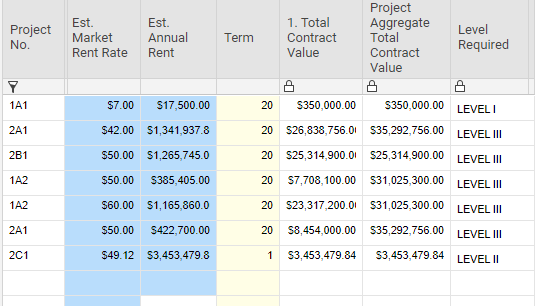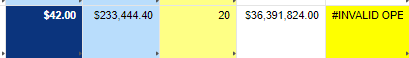# #INVALID OPERATION (SUMIF/SUMIFS to blame?)

The formula I'm trying to fix was not reporting our information correctly so I built a new formula in a test environment and it appeared to be working as intended only for me to find that when applying it in a live setting, the result was constant #INVALID OPERATION errors.

The formula is this:

=IF([Est. Annual Rent]@row = 0, " ", IF(SUMIF([Project No.]:[Project No.], [Project No.]@row, [Est. Annual Rent]:[Est. Annual Rent]) < 280000, "LEVEL I", IF([Project Aggregate Total Contract Value]@row < 10000000, "LEVEL II", IF([Project Aggregate Total Contract Value]@row > 10000000, "LEVEL III"))))

I made another version two with a modification to the end of the formula to see if that would help (It didn't):

=IF([Est. Annual Rent]@row = 0, "", IF(SUMIF([Project No.]:[Project No.], [Project No.]@row, [Est. Annual Rent]:[Est. Annual Rent]) < 280000, "LEVEL I", IF([Project Aggregate Total Contract Value]@row < 10000000, "LEVEL II", "LEVEL III")))

What I've Tried:

1. In both the testing and live environments, all the column types are Text/Number.
2. I switched the SUMIF to SUMIFS (As well as switching the range/criteria ranges to match what the function calls for)
3. Rewriting the formulas from scratch to ensure syntax wasn't the issue (It still may be)

Screen Shots included to show column structure and errors